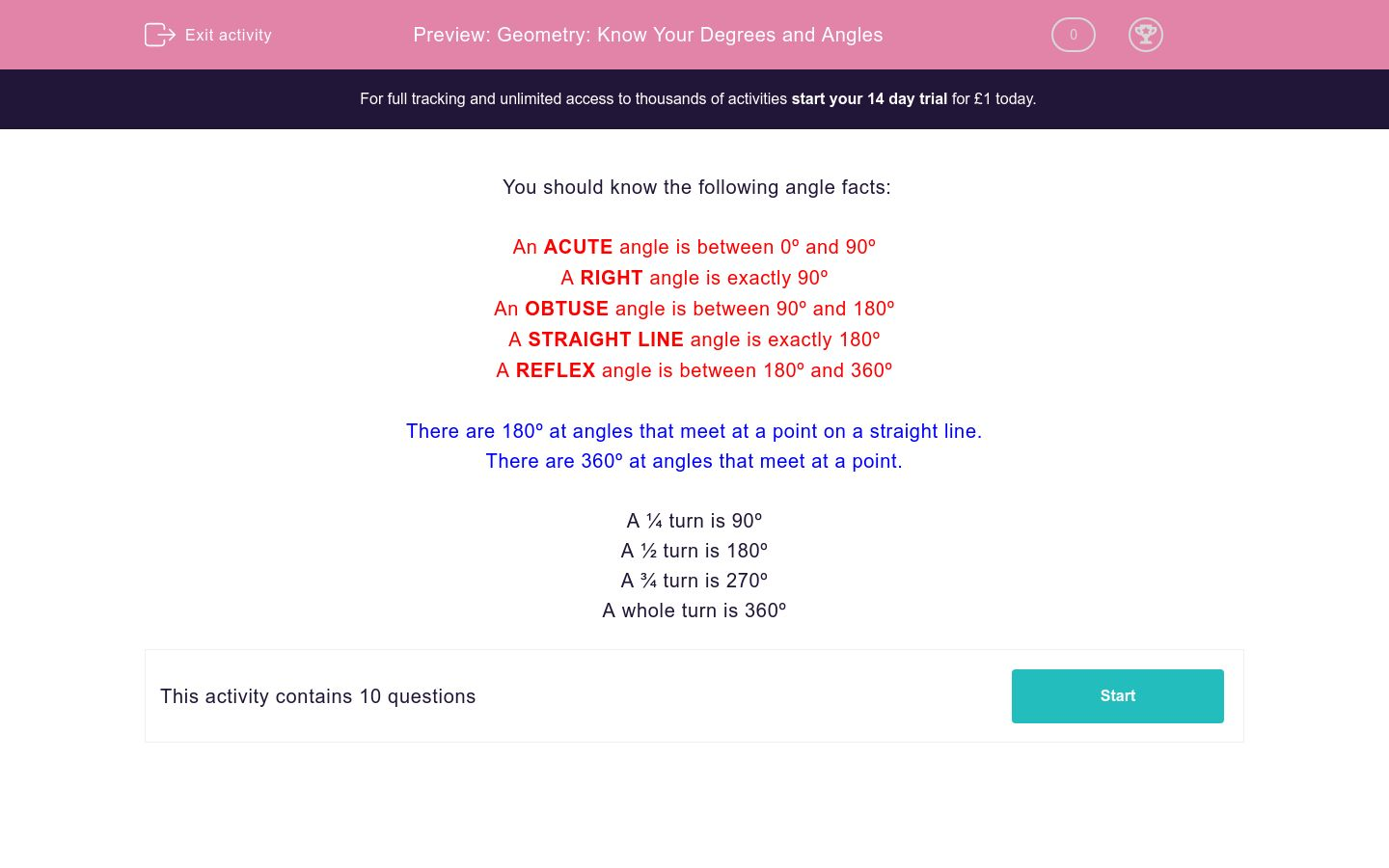# Geometry: Know Your Degrees and Angles

In this worksheet, students answer general questions about angles and learn some facts about shapes.Key stage:  KS 2

Curriculum topic:  Maths and Numerical Reasoning

Curriculum subtopic:  2D Shapes: Circles, Angles and Bearings

Difficulty level:### QUESTION 1 of 10

You should know the following angle facts:

An ACUTE angle is between 0º and 90º

A RIGHT angle is exactly 90º

An OBTUSE angle is between 90º and 180º

A STRAIGHT LINE angle is exactly 180º

A REFLEX angle is between 180º and 360º

There are 180º at angles that meet at a point on a straight line.

There are 360º at angles that meet at a point.

A ¼ turn is 90º

A ½ turn is 180º

A ¾ turn is 270º

A whole turn is 360º

How many degrees are there in a full turn?

How many degrees are there in a right angle?

Complete:

A 59º angle is.....

acute

right-angled

obtuse

a straight line angle

reflex

Complete:

An obtuse angle is.....

less than 90°

90°

between 90° and 180°

180°

between 180° and 360°

Complete:

The angles at a point on a straight line add up to ............ º

Which instrument would you use to draw and angle of 125º ?

a ruler

a set square

a compass

a protractor

Complete:

A reflex angle is.....

less than 90°

90°

between 90° and 180°

180°

between 180° and 360°

Two of the three angles at a point on a straight line are 54º and 85º.

Work out the size of the third angle in degrees.

Two of the three angles around a point are 54º and 85º.

Work out the size of the third angle in degrees.

An angle which is between 170º and 270º could be........

(tick all that apply)

reflex

right-angled

a straight line angle

acute

obtuse

• Question 1

How many degrees are there in a full turn?

360
• Question 2

How many degrees are there in a right angle?

90
• Question 3

Complete:

A 59º angle is.....

acute
• Question 4

Complete:

An obtuse angle is.....

between 90° and 180°
• Question 5

Complete:

The angles at a point on a straight line add up to ............ º

180
• Question 6

Which instrument would you use to draw and angle of 125º ?

a protractor
• Question 7

Complete:

A reflex angle is.....

between 180° and 360°
• Question 8

Two of the three angles at a point on a straight line are 54º and 85º.

Work out the size of the third angle in degrees.

41
• Question 9

Two of the three angles around a point are 54º and 85º.

Work out the size of the third angle in degrees.

221
• Question 10

An angle which is between 170º and 270º could be........

(tick all that apply)

reflex
a straight line angle
obtuse
---- OR ----

Sign up for a £1 trial so you can track and measure your child's progress on this activity.

### What is EdPlace?

We're your National Curriculum aligned online education content provider helping each child succeed in English, maths and science from year 1 to GCSE. With an EdPlace account you’ll be able to track and measure progress, helping each child achieve their best. We build confidence and attainment by personalising each child’s learning at a level that suits them.

Get started#### Similar activities you may like

Start your £1 trial today.
Subscribe from £10/month.